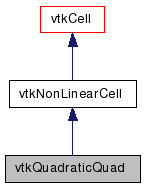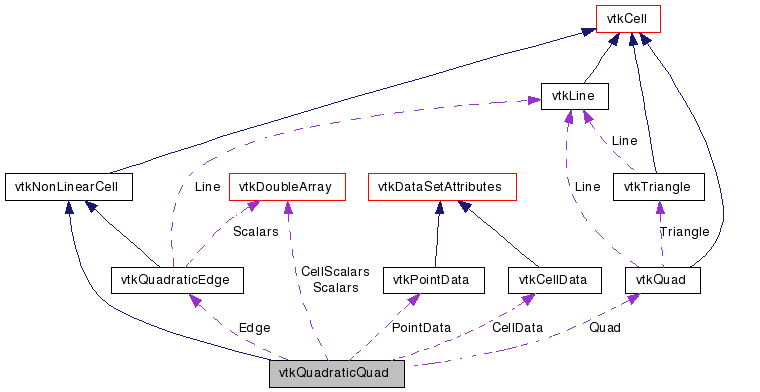`#include <vtkQuadraticQuad.h>`[legend][legend]

## Detailed Description

cell represents a parabolic, 8-node isoparametric quad

vtkQuadraticQuad is a concrete implementation of vtkNonLinearCell to represent a two-dimensional, 8-node isoparametric parabolic quadrilateral element. The interpolation is the standard finite element, quadratic isoparametric shape function. The cell includes a mid-edge node for each of the four edges of the cell. The ordering of the eight points defining the cell are point ids (0-3,4-7) where ids 0-3 define the four corner vertices of the quad; ids 4-7 define the midedge nodes (0,1), (1,2), (2,3), (3,0).

Tests:

static void InterpolationFunctions (double pcoords, double weights)
static void InterpolationDerivs (double pcoords, double derivs)
virtual void InterpolateFunctions (double pcoords, double weights)
virtual void InterpolateDerivs (double pcoords, double derivs)

## Public Types

typedef vtkNonLinearCell Superclass

## Public Member Functions

virtual const char * GetClassName ()
virtual int IsA (const char *type)
void PrintSelf (ostream &os, vtkIndent indent)
int CellBoundary (int subId, double pcoords, vtkIdList *pts)
void Contour (double value, vtkDataArray *cellScalars, vtkIncrementalPointLocator *locator, vtkCellArray *verts, vtkCellArray *lines, vtkCellArray *polys, vtkPointData *inPd, vtkPointData *outPd, vtkCellData *inCd, vtkIdType cellId, vtkCellData *outCd)
int EvaluatePosition (double x, double *closestPoint, int &subId, double pcoords, double &dist2, double *weights)
void EvaluateLocation (int &subId, double pcoords, double x, double *weights)
int Triangulate (int index, vtkIdList *ptIds, vtkPoints *pts)
void Derivatives (int subId, double pcoords, double *values, int dim, double *derivs)
virtual double * GetParametricCoords ()
int GetParametricCenter (double pcoords)
int GetCellType ()
int GetCellDimension ()
int GetNumberOfEdges ()
int GetNumberOfFaces ()
vtkCellGetEdge (int)
vtkCellGetFace (int)
void Clip (double value, vtkDataArray *cellScalars, vtkIncrementalPointLocator *locator, vtkCellArray *polys, vtkPointData *inPd, vtkPointData *outPd, vtkCellData *inCd, vtkIdType cellId, vtkCellData *outCd, int insideOut)
int IntersectWithLine (double p1, double p2, double tol, double &t, double x, double pcoords, int &subId)

## Static Public Member Functions

static int IsTypeOf (const char *type)

## Protected Member Functions

void Subdivide (double *weights)
void InterpolateAttributes (vtkPointData *inPd, vtkCellData *inCd, vtkIdType cellId, vtkDataArray *cellScalars)

## Protected Attributes

vtkPointDataPointData
vtkDoubleArrayScalars
vtkCellDataCellData
vtkDoubleArrayCellScalars

## Member Typedef Documentation

Reimplemented from vtkNonLinearCell.

## Constructor & Destructor Documentation

 vtkQuadraticQuad::vtkQuadraticQuad ( ) ` [protected]`

 vtkQuadraticQuad::~vtkQuadraticQuad ( ) ` [protected]`

## Member Function Documentation

 static vtkQuadraticQuad* vtkQuadraticQuad::New ( ) ` [static]`

Create an object with Debug turned off, modified time initialized to zero, and reference counting on.

Reimplemented from vtkObject.

 virtual const char* vtkQuadraticQuad::GetClassName ( ) ` [virtual]`

Reimplemented from vtkNonLinearCell.

 static int vtkQuadraticQuad::IsTypeOf ( const char * name ) ` [static]`

Return 1 if this class type is the same type of (or a subclass of) the named class. Returns 0 otherwise. This method works in combination with vtkTypeMacro found in vtkSetGet.h.

Reimplemented from vtkNonLinearCell.

 virtual int vtkQuadraticQuad::IsA ( const char * name ) ` [virtual]`

Return 1 if this class is the same type of (or a subclass of) the named class. Returns 0 otherwise. This method works in combination with vtkTypeMacro found in vtkSetGet.h.

Reimplemented from vtkNonLinearCell.

 static vtkQuadraticQuad* vtkQuadraticQuad::SafeDownCast ( vtkObject * o ) ` [static]`

Reimplemented from vtkNonLinearCell.

 void vtkQuadraticQuad::PrintSelf ( ostream & os, vtkIndent indent ) ` [virtual]`

Methods invoked by print to print information about the object including superclasses. Typically not called by the user (use Print() instead) but used in the hierarchical print process to combine the output of several classes.

Reimplemented from vtkNonLinearCell.

 int vtkQuadraticQuad::GetCellType ( ) ` [inline, virtual]`

Implement the vtkCell API. See the vtkCell API for descriptions of these methods.

Implements vtkCell.

 int vtkQuadraticQuad::GetCellDimension ( ) ` [inline, virtual]`

Implement the vtkCell API. See the vtkCell API for descriptions of these methods.

Implements vtkCell.

 int vtkQuadraticQuad::GetNumberOfEdges ( ) ` [inline, virtual]`

Implement the vtkCell API. See the vtkCell API for descriptions of these methods.

Implements vtkCell.

 int vtkQuadraticQuad::GetNumberOfFaces ( ) ` [inline, virtual]`

Implement the vtkCell API. See the vtkCell API for descriptions of these methods.

Implements vtkCell.

 vtkCell* vtkQuadraticQuad::GetEdge ( int ) ` [virtual]`

Implement the vtkCell API. See the vtkCell API for descriptions of these methods.

Implements vtkCell.

 vtkCell* vtkQuadraticQuad::GetFace ( int ) ` [inline, virtual]`

Implement the vtkCell API. See the vtkCell API for descriptions of these methods.

Implements vtkCell.

 int vtkQuadraticQuad::CellBoundary ( int subId, double pcoords, vtkIdList * pts ) ` [virtual]`

Given parametric coordinates of a point, return the closest cell boundary, and whether the point is inside or outside of the cell. The cell boundary is defined by a list of points (pts) that specify a face (3D cell), edge (2D cell), or vertex (1D cell). If the return value of the method is != 0, then the point is inside the cell.

Implements vtkCell.

 void vtkQuadraticQuad::Contour ( double value, vtkDataArray * cellScalars, vtkIncrementalPointLocator * locator, vtkCellArray * verts, vtkCellArray * lines, vtkCellArray * polys, vtkPointData * inPd, vtkPointData * outPd, vtkCellData * inCd, vtkIdType cellId, vtkCellData * outCd ) ` [virtual]`

Generate contouring primitives. The scalar list cellScalars are scalar values at each cell point. The point locator is essentially a points list that merges points as they are inserted (i.e., prevents duplicates). Contouring primitives can be vertices, lines, or polygons. It is possible to interpolate point data along the edge by providing input and output point data - if outPd is NULL, then no interpolation is performed. Also, if the output cell data is non-NULL, the cell data from the contoured cell is passed to the generated contouring primitives. (Note: the CopyAllocate() method must be invoked on both the output cell and point data. The cellId refers to the cell from which the cell data is copied.)

Implements vtkCell.

 int vtkQuadraticQuad::EvaluatePosition ( double x, double * closestPoint, int & subId, double pcoords, double & dist2, double * weights ) ` [virtual]`

Given a point x return inside(=1), outside(=0) cell, or (-1) computational problem encountered; evaluate parametric coordinates, sub-cell id (!=0 only if cell is composite), distance squared of point x to cell (in particular, the sub-cell indicated), closest point on cell to x (unless closestPoint is null, in which case, the closest point and dist2 are not found), and interpolation weights in cell. (The number of weights is equal to the number of points defining the cell). Note: on rare occasions a -1 is returned from the method. This means that numerical error has occurred and all data returned from this method should be ignored. Also, inside/outside is determine parametrically. That is, a point is inside if it satisfies parametric limits. This can cause problems for cells of topological dimension 2 or less, since a point in 3D can project onto the cell within parametric limits but be "far" from the cell. Thus the value dist2 may be checked to determine true in/out.

Implements vtkCell.

 void vtkQuadraticQuad::EvaluateLocation ( int & subId, double pcoords, double x, double * weights ) ` [virtual]`

Determine global coordinate (x) from subId and parametric coordinates. Also returns interpolation weights. (The number of weights is equal to the number of points in the cell.)

Implements vtkCell.

 int vtkQuadraticQuad::Triangulate ( int index, vtkIdList * ptIds, vtkPoints * pts ) ` [virtual]`

Generate simplices of proper dimension. If cell is 3D, tetrahedron are generated; if 2D triangles; if 1D lines; if 0D points. The form of the output is a sequence of points, each n+1 points (where n is topological cell dimension) defining a simplex. The index is a parameter that controls which triangulation to use (if more than one is possible). If numerical degeneracy encountered, 0 is returned, otherwise 1 is returned. This method does not insert new points: all the points that define the simplices are the points that define the cell.

Implements vtkCell.

 void vtkQuadraticQuad::Derivatives ( int subId, double pcoords, double * values, int dim, double * derivs ) ` [virtual]`

Compute derivatives given cell subId and parametric coordinates. The values array is a series of data value(s) at the cell points. There is a one-to-one correspondence between cell point and data value(s). Dim is the number of data values per cell point. Derivs are derivatives in the x-y-z coordinate directions for each data value. Thus, if computing derivatives for a scalar function in a hexahedron, dim=1, 8 values are supplied, and 3 deriv values are returned (i.e., derivatives in x-y-z directions). On the other hand, if computing derivatives of velocity (vx,vy,vz) dim=3, 24 values are supplied ((vx,vy,vz)1, (vx,vy,vz)2, ....()8), and 9 deriv values are returned ((d(vx)/dx),(d(vx)/dy),(d(vx)/dz), (d(vy)/dx),(d(vy)/dy), (d(vy)/dz), (d(vz)/dx),(d(vz)/dy),(d(vz)/dz)).

Implements vtkCell.

 virtual double* vtkQuadraticQuad::GetParametricCoords ( ) ` [virtual]`

Return a contiguous array of parametric coordinates of the points defining this cell. In other words, (px,py,pz, px,py,pz, etc..) The coordinates are ordered consistent with the definition of the point ordering for the cell. This method returns a non-NULL pointer when the cell is a primary type (i.e., IsPrimaryCell() is true). Note that 3D parametric coordinates are returned no matter what the topological dimension of the cell.

Reimplemented from vtkCell.

 void vtkQuadraticQuad::Clip ( double value, vtkDataArray * cellScalars, vtkIncrementalPointLocator * locator, vtkCellArray * polys, vtkPointData * inPd, vtkPointData * outPd, vtkCellData * inCd, vtkIdType cellId, vtkCellData * outCd, int insideOut ) ` [virtual]`

Clip this quadratic quad using scalar value provided. Like contouring, except that it cuts the quad to produce linear triangles.

Implements vtkCell.

 int vtkQuadraticQuad::IntersectWithLine ( double p1, double p2, double tol, double & t, double x, double pcoords, int & subId ) ` [virtual]`

Line-edge intersection. Intersection has to occur within [0,1] parametric coordinates and with specified tolerance.

Implements vtkCell.

 int vtkQuadraticQuad::GetParametricCenter ( double pcoords ) ` [inline, virtual]`

Return the center of the pyramid in parametric coordinates.

Reimplemented from vtkCell.

 static void vtkQuadraticQuad::InterpolationFunctions ( double pcoords, double weights ) ` [static]`

 static void vtkQuadraticQuad::InterpolationDerivs ( double pcoords, double derivs ) ` [static]`

 virtual void vtkQuadraticQuad::InterpolateFunctions ( double pcoords, double weights ) ` [inline, virtual]`

Deprecated:

 virtual void vtkQuadraticQuad::InterpolateDerivs ( double pcoords, double derivs ) ` [inline, virtual]`

Deprecated:

 void vtkQuadraticQuad::Subdivide ( double * weights ) ` [protected]`

 void vtkQuadraticQuad::InterpolateAttributes ( vtkPointData * inPd, vtkCellData * inCd, vtkIdType cellId, vtkDataArray * cellScalars ) ` [protected]`

## Member Data Documentation

 vtkQuadraticEdge* vtkQuadraticQuad::Edge` [protected]`

 vtkQuad* vtkQuadraticQuad::Quad` [protected]`

 vtkPointData* vtkQuadraticQuad::PointData` [protected]`

 vtkDoubleArray* vtkQuadraticQuad::Scalars` [protected]`

 vtkCellData* vtkQuadraticQuad::CellData` [protected]`
 vtkDoubleArray* vtkQuadraticQuad::CellScalars` [protected]`
Generated on Wed Aug 24 12:01:13 2011 for VTK by1.5.6